Confidence Intervals

# 39 A Confidence Interval for a Population Standard Deviation, Known or Large Sample Size

A confidence interval for a population mean with a known population standard deviation is based on the conclusion of the Central Limit Theorem that the sampling distribution of the sample means follow an approximately normal distribution.

### Calculating the Confidence Interval

Consider the standardizing formula for the sampling distribution developed in the discussion of the Central Limit Theorem: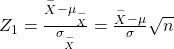Notice that µ is substituted for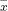because we know that the expected value ofis µ from the Central Limit theorem and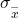is replaced with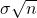, also from the Central Limit Theorem.

In this formula we know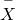,and n, the sample size. (In actuality we do not know the population standard deviation, but we do have a point estimate for it, s, from the sample we took. More on this later.) What we do not know is μ or Z1. We can solve for either one of these in terms of the other. Solving for μ in terms of Z1 gives: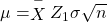Remembering that the Central Limit Theorem tells us that the distribution of the‘s, the sampling distribution for means, is normal, and that the normal distribution is symmetrical, we can rearrange terms thus: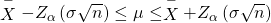This is the formula for a confidence interval for the mean of a population.

Notice that Zα has been substituted for Z1 in this equation. This is where a choice must be made by the statistician. The analyst must decide the level of confidence they wish to impose on the confidence interval. α is the probability that the interval will not contain the true population mean. The confidence level is defined as (1-α). Zα is the number of standard deviationslies from the mean with a certain probability. If we chose Zα = 1.96 we are asking for the 95% confidence interval because we are setting the probability that the true mean lies within the range at 0.95. If we set Zα at 1.64 we are asking for the 90% confidence interval because we have set the probability at 0.90. These numbers can be verified by consulting the Standard Normal table. Divide either 0.95 or 0.90 in half and find that probability inside the body of the table. Then read on the top and left margins the number of standard deviations it takes to get this level of probability.

In reality, we can set whatever level of confidence we desire simply by changing the Zα value in the formula. It is the analyst’s choice. Common convention in Economics and most social sciences sets confidence intervals at either 90, 95, or 99 percent levels. Levels less than 90% are considered of little value. The level of confidence of a particular interval estimate is called by (1-α).

A good way to see the development of a confidence interval is to graphically depict the solution to a problem requesting a confidence interval. This is presented in (Figure) for the example in the introduction concerning the number of downloads from iTunes. That case was for a 95% confidence interval, but other levels of confidence could have just as easily been chosen depending on the need of the analyst. However, the level of confidence MUST be pre-set and not subject to revision as a result of the calculations.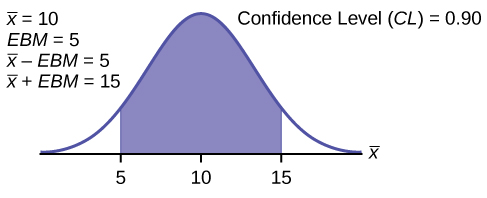For this example, let’s say we know that the actual population mean number of iTunes downloads is 2.1. The true population mean falls within the range of the 95% confidence interval. There is absolutely nothing to guarantee that this will happen. Further, if the true mean falls outside of the interval we will never know it. We must always remember that we will never ever know the true mean. Statistics simply allows us, with a given level of probability (confidence), to say that the true mean is within the range calculated. This is what was called in the introduction, the “level of ignorance admitted”.

### Changing the Confidence Level or Sample Size

Here again is the formula for a confidence interval for an unknown population mean assuming we know the population standard deviation:It is clear that the confidence interval is driven by two things, the chosen level of confidence,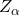, and the standard deviation of the sampling distribution. The Standard deviation of the sampling distribution is further affected by two things, the standard deviation of the population and the sample size we chose for our data. Here we wish to examine the effects of each of the choices we have made on the calculated confidence interval, the confidence level and the sample size.

For a moment we should ask just what we desire in a confidence interval. Our goal was to estimate the population mean from a sample. We have forsaken the hope that we will ever find the true population mean, and population standard deviation for that matter, for any case except where we have an extremely small population and the cost of gathering the data of interest is very small. In all other cases we must rely on samples. With the Central Limit Theorem we have the tools to provide a meaningful confidence interval with a given level of confidence, meaning a known probability of being wrong. By meaningful confidence interval we mean one that is useful. Imagine that you are asked for a confidence interval for the ages of your classmates. You have taken a sample and find a mean of 19.8 years. You wish to be very confident so you report an interval between 9.8 years and 29.8 years. This interval would certainly contain the true population mean and have a very high confidence level. However, it hardly qualifies as meaningful. The very best confidence interval is narrow while having high confidence. There is a natural tension between these two goals. The higher the level of confidence the wider the confidence interval as the case of the students’ ages above. We can see this tension in the equation for the confidence interval.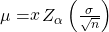The confidence interval will increase in width as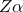increases,increases as the level of confidence increases. There is a tradeoff between the level of confidence and the width of the interval. Now let’s look at the formula again and we see that the sample size also plays an important role in the width of the confidence interval. The sample sized,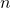, shows up in the denominator of the standard deviation of the sampling distribution. As the sample size increases, the standard deviation of the sampling distribution decreases and thus the width of the confidence interval, while holding constant the level of confidence. This relationship was demonstrated in (Figure). Again we see the importance of having large samples for our analysis although we then face a second constraint, the cost of gathering data.

### Calculating the Confidence Interval: An Alternative Approach

Another way to approach confidence intervals is through the use of something called the Error Bound. The Error Bound gets its name from the recognition that it provides the boundary of the interval derived from the standard error of the sampling distribution. In the equations above it is seen that the interval is simply the estimated mean, sample mean, plus or minus something. That something is the Error Bound and is driven by the probability we desire to maintain in our estimate,, times the standard deviation of the sampling distribution. The Error Bound for a mean is given the name, Error Bound Mean, or EBM.

To construct a confidence interval for a single unknown population mean μ, where the population standard deviation is known, we need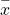as an estimate for μ and we need the margin of error. Here, the margin of error (EBM) is called the error bound for a population mean (abbreviated EBM). The sample meanis the point estimate of the unknown population mean μ.

The confidence interval estimate will have the form:

(point estimate – error bound, point estimate + error bound) or, in symbols,(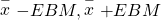)

The mathematical formula for this confidence interval is:The margin of error (EBM) depends on the confidence level (abbreviated CL). The confidence level is often considered the probability that the calculated confidence interval estimate will contain the true population parameter. However, it is more accurate to state that the confidence level is the percent of confidence intervals that contain the true population parameter when repeated samples are taken. Most often, it is the choice of the person constructing the confidence interval to choose a confidence level of 90% or higher because that person wants to be reasonably certain of his or her conclusions.

There is another probability called alpha (α). α is related to the confidence level, CL. α is the probability that the interval does not contain the unknown population parameter.
Mathematically, 1 – α = CL.

A confidence interval for a population mean with a known standard deviation is based on the fact that the sampling distribution of the sample means follow an approximately normal distribution. Suppose that our sample has a mean of= 10, and we have constructed the 90% confidence interval (5, 15) where EBM = 5.

To get a 90% confidence interval, we must include the central 90% of the probability of the normal distribution. If we include the central 90%, we leave out a total of α = 10% in both tails, or 5% in each tail, of the normal distribution.To capture the central 90%, we must go out 1.645 standard deviations on either side of the calculated sample mean. The value 1.645 is the z-score from a standard normal probability distribution that puts an area of 0.90 in the center, an area of 0.05 in the far left tail, and an area of 0.05 in the far right tail.

It is important that the standard deviation used must be appropriate for the parameter we are estimating, so in this section we need to use the standard deviation that applies to the sampling distribution for means which we studied with the Central Limit Theorem and is,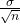.

#### Calculating the Confidence Interval Using EMB

To construct a confidence interval estimate for an unknown population mean, we need data from a random sample. The steps to construct and interpret the confidence interval are:

• Calculate the sample meanfrom the sample data. Remember, in this section we know the population standard deviation σ.
• Find the z-score from the standard normal table that corresponds to the confidence level desired.
• Calculate the error bound EBM.
• Construct the confidence interval.
• Write a sentence that interprets the estimate in the context of the situation in the problem.

We will first examine each step in more detail, and then illustrate the process with some examples.

#### Finding the z-score for the Stated Confidence Level

When we know the population standard deviation σ, we use a standard normal distribution to calculate the error bound EBM and construct the confidence interval. We need to find the value of z that puts an area equal to the confidence level (in decimal form) in the middle of the standard normal distribution Z ~ N(0, 1).

The confidence level, CL, is the area in the middle of the standard normal distribution. CL = 1 – α, so α is the area that is split equally between the two tails. Each of the tails contains an area equal to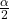.

The z-score that has an area to the right ofis denoted by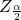.

For example, when CL = 0.95, α = 0.05 and= 0.025; we write=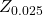.

The area to the right ofis 0.025 and the area to the left ofis 1 – 0.025 = 0.975.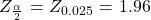, using a standard normal probability table. We will see later that we can use a different probability table, the Student’s t-distribution, for finding the number of standard deviations of commonly used levels of confidence.

#### Calculating the Error Bound (EBM)

The error bound formula for an unknown population mean μ when the population standard deviation σ is known is

• EBM =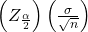#### Constructing the Confidence Interval

• The confidence interval estimate has the format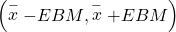or the formula:The graph gives a picture of the entire situation.

CL ++= CL + α = 1.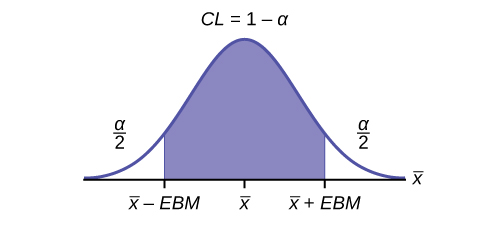Suppose we are interested in the mean scores on an exam. A random sample of 36 scores is taken and gives a sample mean (sample mean score) of 68 (= 68). In this example we have the unusual knowledge that the population standard deviation is 3 points. Do not count on knowing the population parameters outside of textbook examples. Find a confidence interval estimate for the population mean exam score (the mean score on all exams).

Find a 90% confidence interval for the true (population) mean of statistics exam scores.

• The solution is shown step-by-step.

To find the confidence interval, you need the sample mean,, and the EBM.

•= 68
• EBM =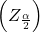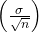• σ = 3; n = 36; The confidence level is 90% (CL = 0.90)

CL = 0.90 so α = 1 – CL = 1 – 0.90 = 0.10= 0.05The area to the right of Z0.05 is 0.05 and the area to the left of Z0.05 is 1 – 0.05 = 0.95.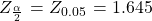This can be found using a computer, or using a probability table for the standard normal distribution. Because the common levels of confidence in the social sciences are 90%, 95% and 99% it will not be long until you become familiar with the numbers , 1.645, 1.96, and 2.56

EBM = (1.645)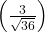= 0.8225EBM = 68 – 0.8225 = 67.1775+ EBM = 68 + 0.8225 = 68.8225

The 90% confidence interval is (67.1775, 68.8225).

InterpretationWe estimate with 90% confidence that the true population mean exam score for all statistics students is between 67.18 and 68.82.

Suppose we change the original problem in (Figure) by using a 95% confidence level. Find a 95% confidence interval for the true (population) mean statistics exam score.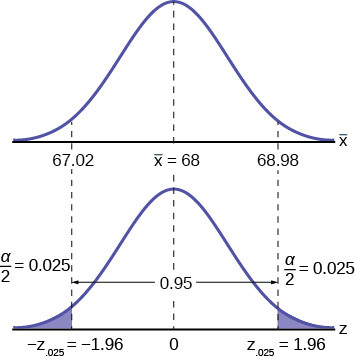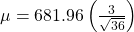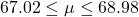σ = 3; n = 36; The confidence level is 95% (CL = 0.95).

CL = 0.95 so α = 1 – CL = 1 – 0.95 = 0.05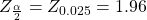Notice that the EBM is larger for a 95% confidence level in the original problem.

Comparing the resultsThe 90% confidence interval is (67.18, 68.82). The 95% confidence interval is (67.02, 68.98). The 95% confidence interval is wider. If you look at the graphs, because the area 0.95 is larger than the area 0.90, it makes sense that the 95% confidence interval is wider. To be more confident that the confidence interval actually does contain the true value of the population mean for all statistics exam scores, the confidence interval necessarily needs to be wider. This demonstrates a very important principle of confidence intervals. There is a trade off between the level of confidence and the width of the interval. Our desire is to have a narrow confidence interval, huge wide intervals provide little information that is useful. But we would also like to have a high level of confidence in our interval. This demonstrates that we cannot have both.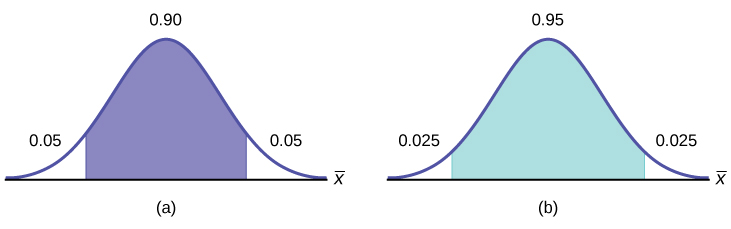Summary: Effect of Changing the Confidence Level
• Increasing the confidence level makes the confidence interval wider.
• Decreasing the confidence level makes the confidence interval narrower.

And again here is the formula for a confidence interval for an unknown mean assuming we have the population standard deviation:The standard deviation of the sampling distribution was provided by the Central Limit Theorem as. While we infrequently get to choose the sample size it plays an important role in the confidence interval. Because the sample size is in the denominator of the equation, asincreases it causes the standard deviation of the sampling distribution to idecrease and thus the width of the confidence interval to decrease. We have met this before as we reviewed the effects of sample size on the Central Limit Theorem. There we saw that asincreases the sampling distribution narrows until in the limit it collapses on the true population mean.

Suppose we change the original problem in (Figure) to see what happens to the confidence interval if the sample size is changed.

Leave everything the same except the sample size. Use the original 90% confidence level. What happens to the confidence interval if we increase the sample size and use n = 100 instead of n = 36? What happens if we decrease the sample size to n = 25 instead of n = 36?

Solution A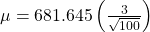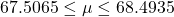If we increase the sample size n to 100, we decrease the width of the confidence interval relative to the original sample size of 36 observations.

Solution B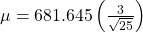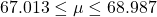If we decrease the sample size n to 25, we increase the width of the confidence interval by comparison to the original sample size of 36 observations.

Summary: Effect of Changing the Sample Size
• Increasing the sample size makes the confidence interval narrower.
• Decreasing the sample size makes the confidence interval wider.

We have already seen this effect when we reviewed the effects of changing the size of the sample, n, on the Central Limit Theorem. See (Figure) to see this effect. Before we saw that as the sample size increased the standard deviation of the sampling distribution decreases. This was why we choose the sample mean from a large sample as compared to a small sample, all other things held constant.

Thus far we assumed that we knew the population standard deviation. This will virtually never be the case. We will have the sample standard deviation, s, however. This is a point estimate for the population standard deviation and can be substituted into the formula for confidence intervals for a mean under certain circumstances. We just saw the effect the sample size has on the width of confidence interval and the impact on the sampling distribution for our discussion of the Central Limit Theorem. We can invoke this to substitute the point estimate for the standard deviation if the sample size is large “enough”. Simulation studies indicate that 30 observations or more will be sufficient to eliminate any meaningful bias in the estimated confidence interval.

Spring break can be a very expensive holiday. A sample of 80 students is surveyed, and the average amount spent by students on travel and beverages is ?593.84. The sample standard deviation is approximately ?369.34.

Construct a 92% confidence interval for the population mean amount of money spent by spring breakers.

We begin with the confidence interval for a mean. We use the formula for a mean because the random variable is dollars spent and this is a continuous random variable. The point estimate for the population standard deviation, s, has been substituted for the true population standard deviation because with 80 observations there is no concern for bias in the estimate of the confidence interval.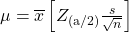Substituting the values into the formula, we have: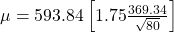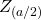is found on the standard normal table by looking up 0.46 in the body of the table and finding the number of standard deviations on the side and top of the table; 1.75. The solution for the interval is thus: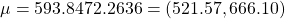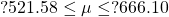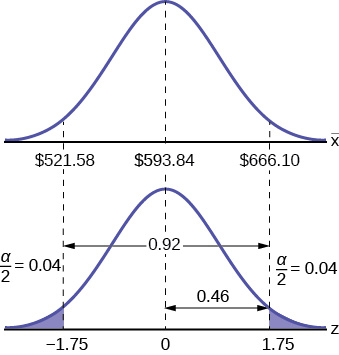### References

“American Fact Finder.” U.S. Census Bureau. Available online at http://factfinder2.census.gov/faces/nav/jsf/pages/searchresults.xhtml?refresh=t (accessed July 2, 2013).

“Disclosure Data Catalog: Candidate Summary Report 2012.” U.S. Federal Election Commission. Available online at http://www.fec.gov/data/index.jsp (accessed July 2, 2013).

“Headcount Enrollment Trends by Student Demographics Ten-Year Fall Trends to Most Recently Completed Fall.” Foothill De Anza Community College District. Available online at http://research.fhda.edu/factbook/FH_Demo_Trends/FoothillDemographicTrends.htm (accessed September 30,2013).

Kuczmarski, Robert J., Cynthia L. Ogden, Shumei S. Guo, Laurence M. Grummer-Strawn, Katherine M. Flegal, Zuguo Mei, Rong Wei, Lester R. Curtin, Alex F. Roche, Clifford L. Johnson. “2000 CDC Growth Charts for the United States: Methods and Development.” Centers for Disease Control and Prevention. Available online at http://www.cdc.gov/growthcharts/2000growthchart-us.pdf (accessed July 2, 2013).

La, Lynn, Kent German. “Cell Phone Radiation Levels.” c|net part of CBX Interactive Inc. Available online at http://reviews.cnet.com/cell-phone-radiation-levels/ (accessed July 2, 2013).

“Mean Income in the Past 12 Months (in 2011 Inflaction-Adjusted Dollars): 2011 American Community Survey 1-Year Estimates.” American Fact Finder, U.S. Census Bureau. Available online at http://factfinder2.census.gov/faces/tableservices/jsf/pages/productview.xhtml?pid=ACS_11_1YR_S1902&prodType=table (accessed July 2, 2013).

“Metadata Description of Candidate Summary File.” U.S. Federal Election Commission. Available online at http://www.fec.gov/finance/disclosure/metadata/metadataforcandidatesummary.shtml (accessed July 2, 2013).

“National Health and Nutrition Examination Survey.” Centers for Disease Control and Prevention. Available online at http://www.cdc.gov/nchs/nhanes.htm (accessed July 2, 2013).

### Formula Review

The general form for a confidence interval for a single population mean, known standard deviation, normal distribution is given byThis formula is used when the population standard deviation is known.

CL = confidence level, or the proportion of confidence intervals created that are expected to contain the true population parameter

α = 1 – CL = the proportion of confidence intervals that will not contain the population parameter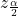= the z-score with the property that the area to the right of the z-score is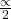this is the z-score used in the calculation of “EBM where α = 1 – CL.

### Key Terms

Confidence Level (CL)
the percent expression for the probability that the confidence interval contains the true population parameter; for example, if the CL = 90%, then in 90 out of 100 samples the interval estimate will enclose the true population parameter.
Error Bound for a Population Mean (EBM)
the margin of error; depends on the confidence level, sample size, and known or estimated population standard deviation.